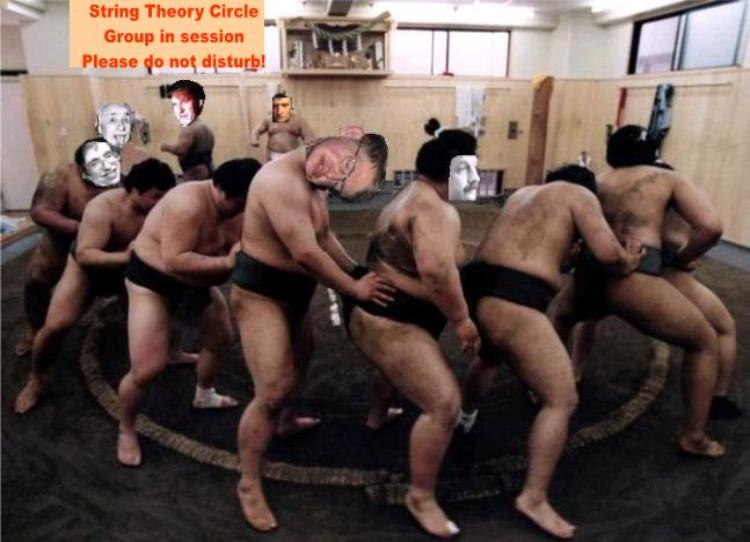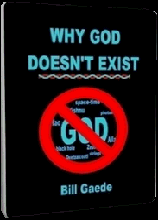So how is it that strings generate gravity?

The first thing you have to be prepared for is that String Theory has no new physical interpretation for gravity. The reason
string theory came about is that the mathematicians were attempting to reconcile General Relativity and Quantum Mechanics.
The status quo is that string theorists still live with another of those famous mathematical dualities. String Theory has TWO
explanations for gravity. One is exactly like the one General Relativity proposes and the other is exactly like the one Quantum
Mechanics proposes. One is based on warped space and the other is based on particles and forces. The idiots known as
string theorists haven't unified absolutely anything! Strings generate gravity no differently than GR and QM. In fact, String
Theory suffers from the same ailment as its parents. ST is just your standard Mathematical Physics irrationalities
(i.e., inconsistent use of terms, object-concept paradoxes, circular definitions, etc.).

1.   Graviton gravity

The dynamic version holds that every particle in Quantum, specifically the graviton, can be described as a vibrating string.
This particle then travels and produces gravity consistent with  negative motion  physics of Quantum Mechanics.

“ So where does gravity come in…? If the string traveling through spacetime is a
closed string, then the spectrum of oscillations includes a particle with 2 units of
spin and zero mass, with the right type of interactions to be the graviton, the
particle that is the carrier of the gravitational force… Where there are gravitons,
then there must be gravity.” 

2.   Ball-on-canvas  gravity

The static version has it that strings coil around and form interlocking links of a grand chain-mail. Space-time is nothing but
an enormous chain-mail. Then, you just follow standard ‘bowling-ball-on- canvasrelativity.

“ One idea is that they are one-dimensional closed ‘strings’ of energy that wiggle
in an 11-dimensional arena. Another idea has gravitons as donut-shaped loops
that interconnect like some enormous chain mail suit.” 

Where is the gravity in string theory…? If a closed string is traveling in a curved
spacetime, then the coordinates of the string in spacetime feel this curvature as
the string propagates. Once again, the answer lies on the string worldsheet. In
order for their [sic] to be a consistent quantum theory in this case, the curved
space in which the string travels must be a solution to the Einstein equations…
Now this is really something!” 

[Yeah. It’s absolutely stunning!]

So assuming that we accept the string hypothesis, Mathematical Physics continues to offer two irrecon- cilable explanations
for gravity. The first explanation says that the Sun throws negative-momentum carrying graviton stones at the Earth to reel it
in. The second one proposes that warped space is made of interlocked links. The Sun weighs this chain-mail ‘downwards’
and the funnel thus created compels the Earth to roll or slide around it. How will the fact that space-time is actually an
enormous chain-mail in any way going to change the gravity well mechanism proposed by relativity? If, instead, the graviton
ball is really a vibrating string, how will this alter the negative-momentum graviton mechanism proposed by Quantum
Mechanics? How do we merge the traveling graviton with a static well that is already there? What does it matter that space-
time is made of particles or of strings or of mathematical ‘brains’ if the constitution of space-time at the micro level has
absolutely nothing to do with the mechanism GR proposes for gravity, which in turn cannot be reconciled with QM’s graviton?

In fact, if String Theory were correct, it would only show that the mathematicians have been either ignorant or lying all these
years about relativity being able to describe and/or explain gravity. GR says that objects weigh down space-time and create
gravity wells to attract other objects. QM says that objects throw graviton stones at each other and transfer negative
momentum. If one is correct, the other one is dead wrong and needs to be discarded. So how did String Theory merge QM
and GR? What was the purpose of this expensive, intergenerational mathematical project if we ended up where we began?Okay, guys! Line up! Glab the fella in flont of you. Let's show Bill how stlings genelate glavity. Watch out you no step on the othel guy's dloppings!
 How strings generate gravity
 Adapted for the Internet from:Why God Doesn't Exist________________________________________________________________________________________